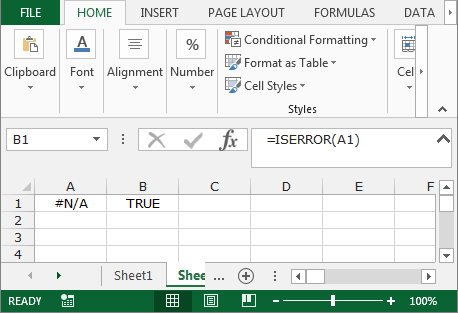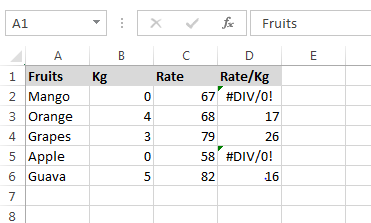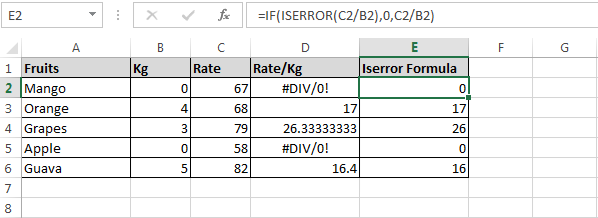ISERROR Function in Microsoft Excel

ISERROR- This function is used for checking whether a value is an error (#N/A, #VALUE!, #REF!, #DIV/0!, #NUM!, #NAME?, OR #NULL!) and returns True or False.

The Syntax of “ISERROR” function:        =ISERROR(Value)Let’s take an example to understand how we can use ISERROR function along with IF function.

We have fruits data in range A1:D6 where Column A contains Fruits Name, Column B contains Kilograms, Column C contains Rate and in column D, we have returned the amount per kilogram.Where kilogram is zero, the formula is giving #DIV/0! Error. We need the formula if the kilogram is zero then result should be 0, else show the rate per kilogram.

• Enter the formula in cell E2.
• =IF(ISERROR(C2/B2),0,C2/B2)
• Press Enter on your keyboard.
• Copy the same formula in the rest of the cells.
• The function will return only value including 0.Using this way, you can ignore the error while performing the calculation in Microsoft Excel.